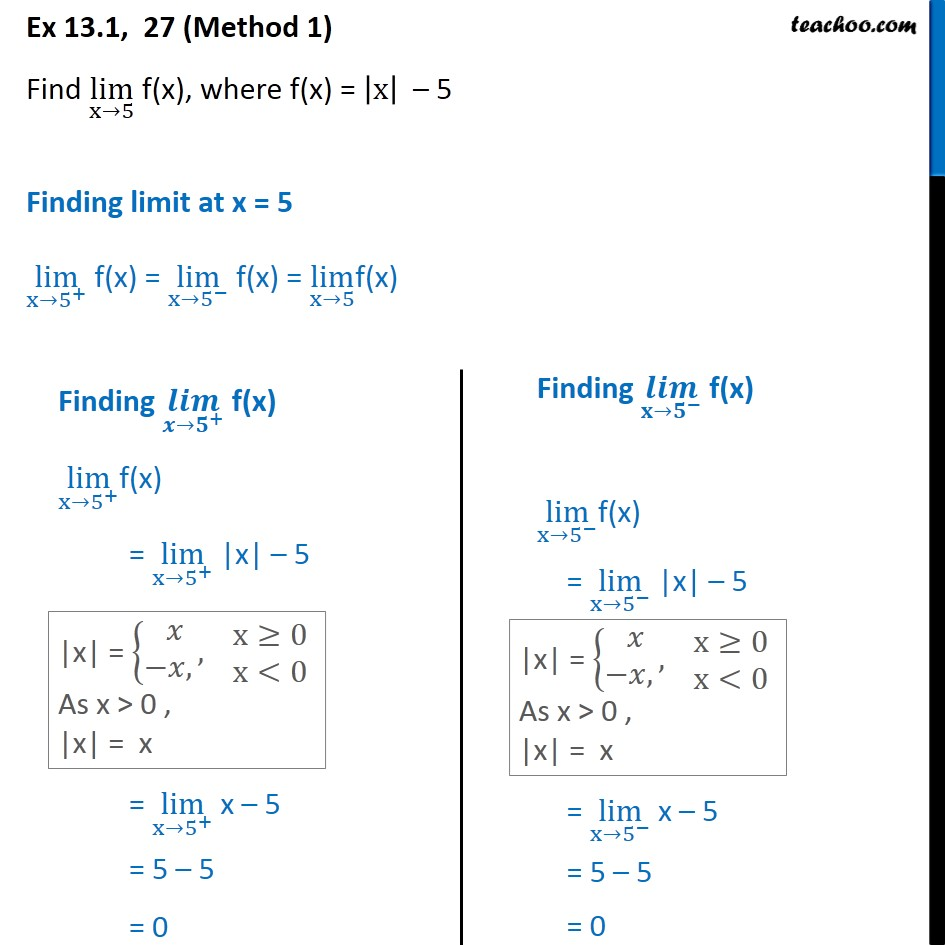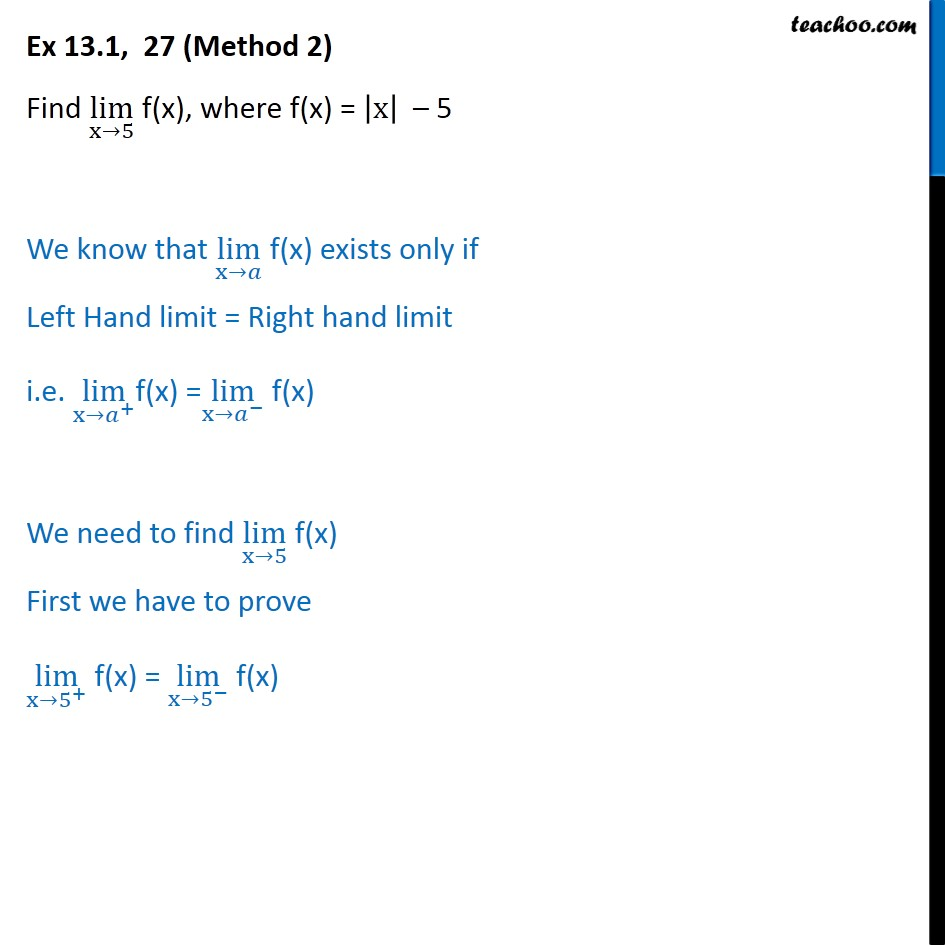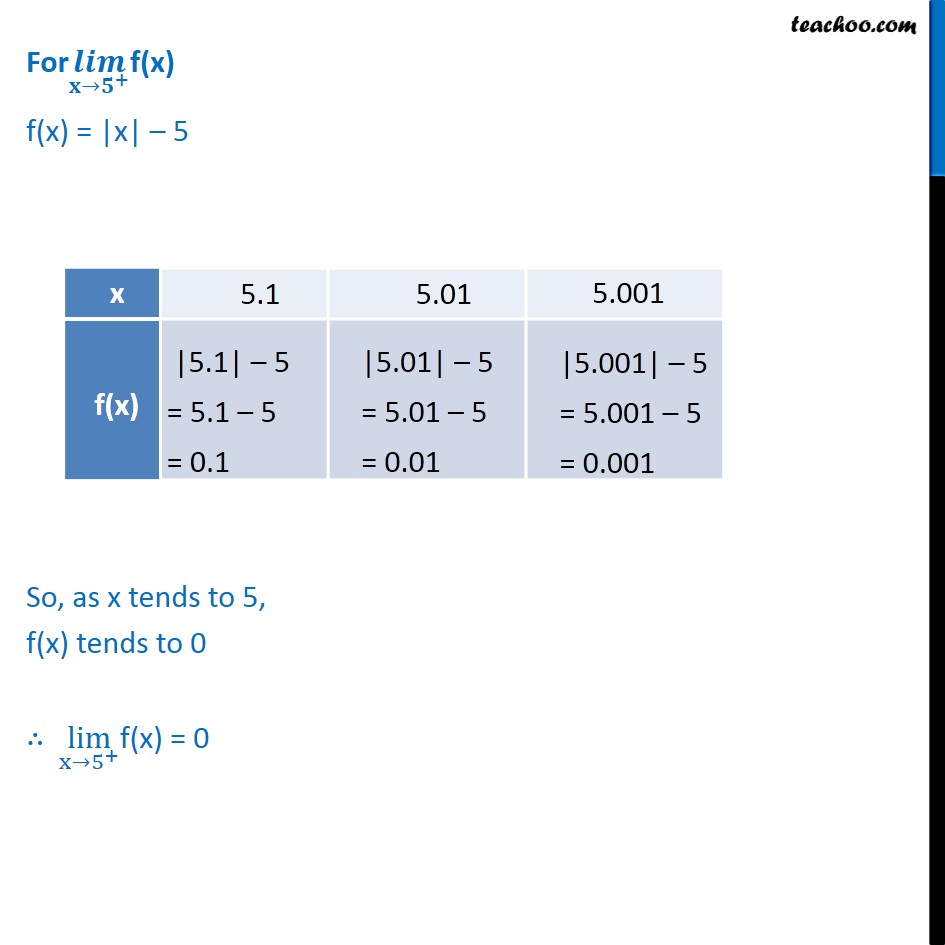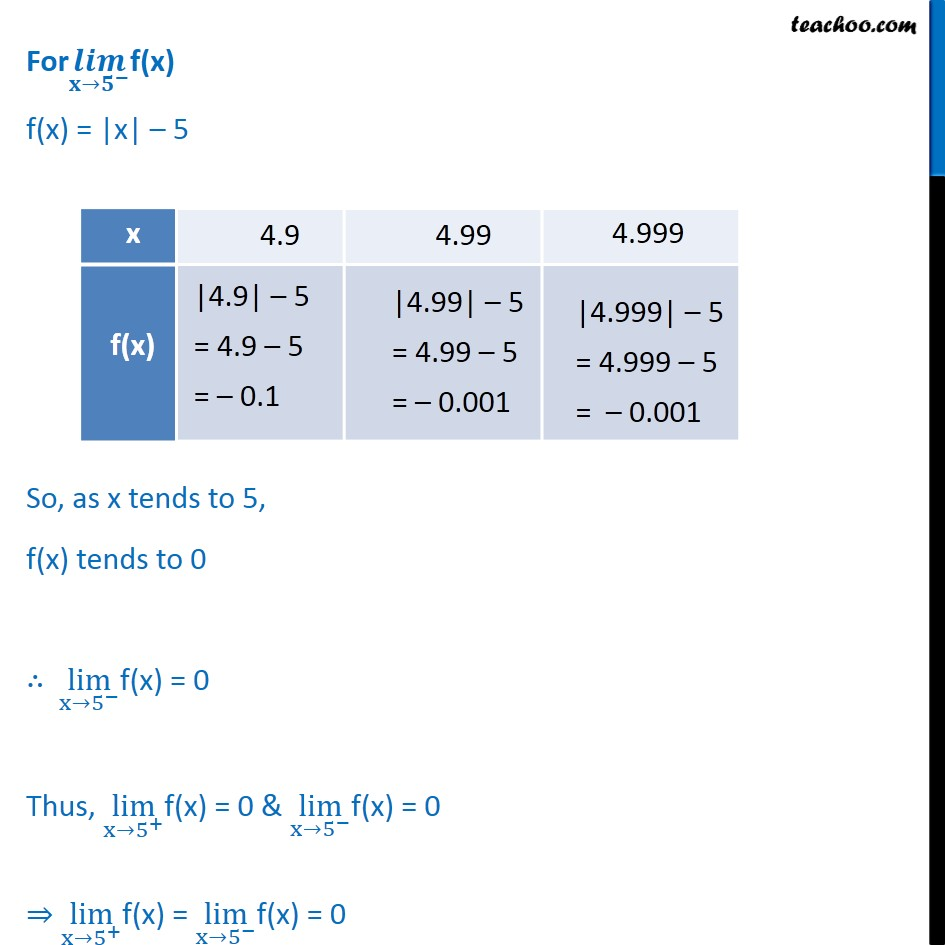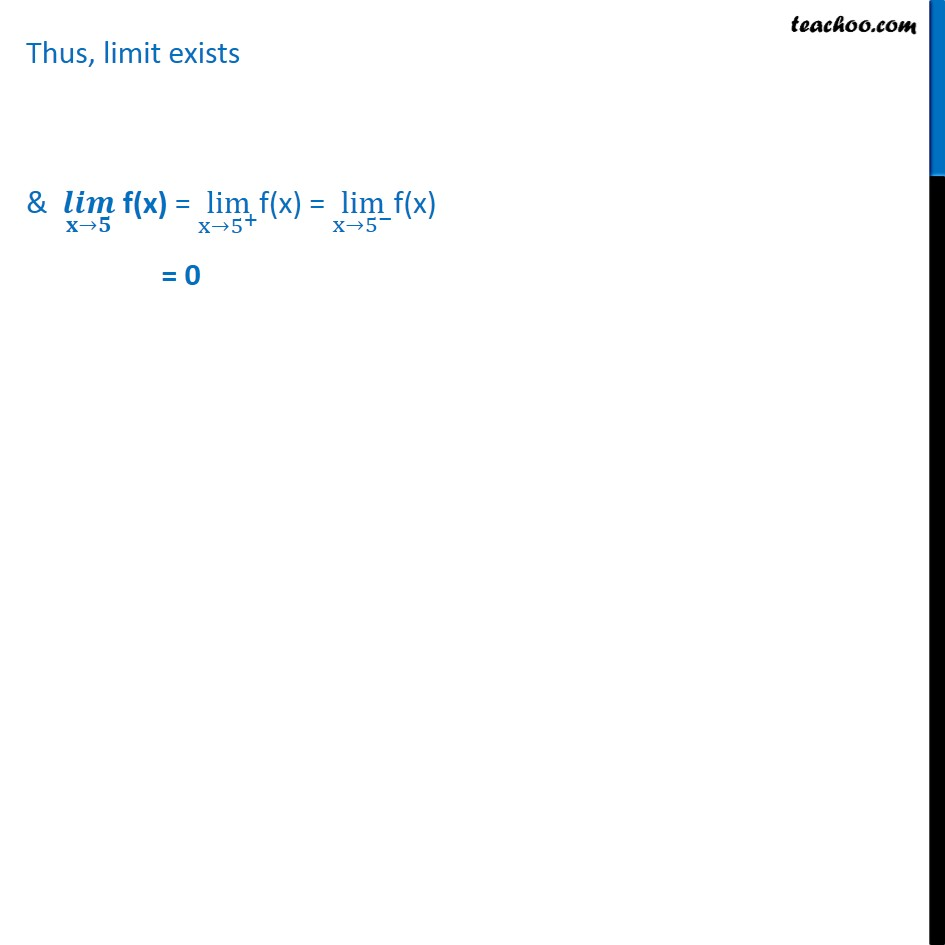1. Chapter 13 Class 11 Limits and Derivatives
2. Concept wise
3. Limits - Limit exists

Transcript

Ex 13.1, 27 (Method 1) Find lim﷮x→5﷯ f(x), where f(x) = x﷯ – 5 Finding limit at x = 5 lim﷮x→ 5﷮+﷯﷯ f(x) = lim﷮x→ 5﷮−﷯﷯ f(x) = lim﷮x→5﷯f(x) ∴ lim﷮ x→5﷮+﷯﷯f(x) = 0 & lim﷮ x→5﷮+﷯﷯f(x) = 0 Now, lim﷮x→5﷯ f(x) = lim﷮ x→5﷮+﷯﷯f(x) = lim﷮ x→5﷮−﷯﷯f(x) = 0 Thus, 𝒍𝒊𝒎﷮𝐱→𝟓﷯ f(x) = 0 Ex 13.1, 27 (Method 2) Find lim﷮x→5﷯ f(x), where f(x) = x﷯ – 5 We know that lim﷮x→𝑎﷯ f(x) exists only if Left Hand limit = Right hand limit i.e. lim﷮ x→𝑎﷮+﷯﷯f(x) = lim﷮ x→𝑎﷮−﷯﷯ f(x) We need to find lim﷮x→5﷯ f(x) First we have to prove lim﷮x→ 5﷮+﷯﷯ f(x) = lim﷮x→ 5﷮−﷯﷯ f(x) For 𝒍𝒊𝒎﷮ 𝐱→𝟓﷮+﷯﷯f(x) f(x) = |x| – 5 So, as x tends to 5, f(x) tends to 0 ∴ lim﷮ x→5﷮+﷯﷯f(x) = 0 For 𝒍𝒊𝒎﷮ 𝐱→𝟓﷮−﷯﷯f(x) f(x) = |x| – 5 So, as x tends to 5, f(x) tends to 0 ∴ lim﷮ x→5﷮−﷯﷯f(x) = 0 Thus, lim﷮ x→5﷮+﷯﷯f(x) = 0 & lim﷮ x→5﷮−﷯﷯f(x) = 0 ⇒ lim﷮ x→5﷮+﷯﷯f(x) = lim﷮ x→5﷮−﷯﷯f(x) = 0 Thus, limit exists & 𝒍𝒊𝒎﷮𝐱→𝟓﷯ f(x) = lim﷮ x→5﷮+﷯﷯f(x) = lim﷮ x→5﷮−﷯﷯f(x) = 0

Limits - Limit exists